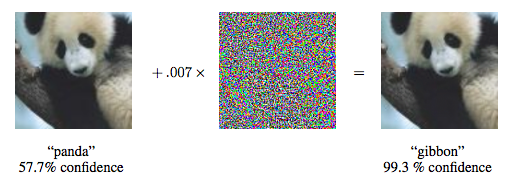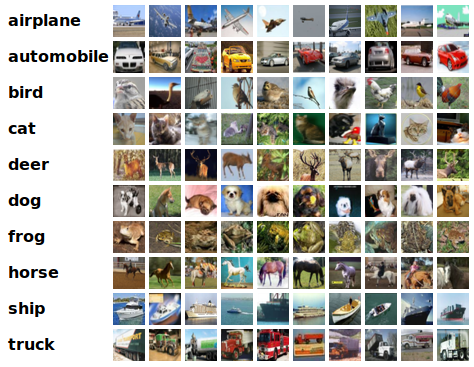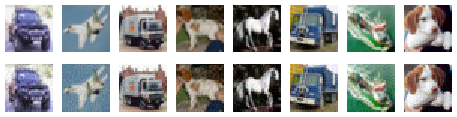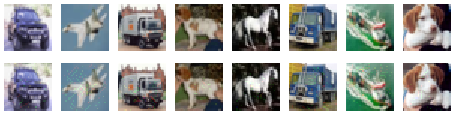# 深度学习的恶意样本实践（Adversarial Example）

## 〇、深度学习的恶意样本（Adversarial Example）## 一、预备工作：CIFAR10与ResNet

Adversarial Example可以攻击各种深度学习应用，包括图像、声音、视频、甚至文本（在NLP领域）等。本文主要以图像的分类/识别任务为例来生成恶意样本。所选择之数据集为经典的CIFAR10（来自文献【１】），如下图所示。该数据集中的图像均为32×32的彩色图像，所有图像都被分成飞机、汽车、鸟、猫等10个类别（分类标签为0~9）。from os.path import abspath
import sys
import os
import tensorflow as tf
sys.path.append(abspath('.'))

import keras
import numpy as np
import pickle

import matplotlib.pyplot as plt
%matplotlib inline

from keras.datasets import cifar10
from keras.models import load_model
from keras.utils import to_categorical

from art.classifiers import KerasClassifier
from art.attacks.fast_gradient import FastGradientMethod
from art.attacks.iterative_method import BasicIterativeMethod
from art.attacks.saliency_map import SaliencyMapMethod
from art.attacks import DeepFool

my_model = load_model('cifar10_ResNet32v1_model.189.h5')

(x_train, y_train), (x_test, y_test) = cifar10.load_data()
x_train = x_train.astype('float32') / 255
x_test = x_test.astype('float32') / 255
x_train_mean = np.mean(x_train, axis=0)
x_train -= x_train_mean
x_test -= x_train_mean

min_ = np.min(x_train)
max_ = np.max(x_train)

num_classes = 10
y_train_vector = keras.utils.to_categorical(y_train, num_classes)
y_test_vector = keras.utils.to_categorical(y_test, num_classes)

scores = my_model.evaluate(x_test, y_test_vector, verbose=1)

print('Test accuracy:', scores)

10000/10000 [==============================] - 4s 375us/step
Test accuracy: 0.9196


len_index = x_test.shape
sum_success = 0

for i in range(len_index):
y_pred = my_model.predict(x_test[i].reshape(1,32,32,3))
if (np.argmax(y_pred) == y_test[i]):
sum_success += 1

print('Test accuracy:', sum_success/10000.0)

Test accuracy: 0.9196


## 二、FGSM

FGSM是一种比较简单的AE生成算法，它由来自谷歌大脑的三位研究者在文献【4】中提出，其中一位作者就是大名鼎鼎的 Goodfellow。Ian Goodfellow因提出了生成对抗网络（GANs）而闻名，他被誉为“GANs之父”，甚至被认为是当前人工智能领域的顶级专家。他还是著名的花书《Deep Learning》的作者。文献【4】中的另外一个作者是Samy Bengio，他除了是深度学习领域的一位知名专家以外，还是新晋图灵奖得主Yoshua Bengio的兄弟。IBM ART工具箱中的FastGradientMethod函数实现了这个算法，下面我们就调用这个函数来对之前训练好的ResNet32网络发动AE攻击。

x_test_1K, y_test_1K = x_test[:1000], y_test_vector[:1000]

classifier = KerasClassifier((min_, max_), model=my_model)

# Craft adversarial samples with FGSM
epsilon = 0.03  # Maximum perturbation
adv_fgsm_crafter = FastGradientMethod(classifier)

x_test_adv_fgsm = adv_fgsm_crafter.generate(x=x_test_1K, eps=epsilon)

scores_adv_test = my_model.evaluate(x_test_adv_fgsm, y_test_1K, verbose=1)
print('Accuracy on Test AEs based on FGSM:', scores_adv_test)

1000/1000 [==============================] - 0s 309us/step
Accuracy on Test AEs based on FGSM: 0.187

fig=plt.figure(figsize=(8, 2))
columns = 8
rows = 2
for i in range(1, 9):
img = x_test[i+8].reshape(32,32,3) + x_train_mean
fig.add_subplot(rows, columns, i)
plt.imshow(img)
plt.axis('off')

for i in range(1, 9):
img_adv = x_test_adv_fgsm[i+8].reshape(32,32,3) + x_train_mean
fig.add_subplot(rows, columns, i+8)
plt.imshow(img_adv)
plt.axis('off')for i in range(9, 17):
y_pred = my_model.predict(x_test[i].reshape(1,32,32,3))
print(np.argmax(y_pred), end = ' ')

print("")
for i in range(9, 17):
y_pred = my_model.predict(x_test_adv_fgsm[i].reshape(1,32,32,3))
print(np.argmax(y_pred), end = ' ')

1 0 9 5 7 9 8 5
9 3 1 4 6 1 6 3 

## 三、IGSM

epsilon=0.015  # Maximum perturbation
stepsize=0.005
adv_igsm_crafter = BasicIterativeMethod(classifier, eps=epsilon, eps_step=stepsize)

x_test_adv_igsm = adv_igsm_crafter.generate(x=x_test_1K)

scores_adv_test = my_model.evaluate(x_test_adv_igsm, y_test_1K, verbose=1)
print('Accuracy on Test AEs based on IGSM:', scores_adv_test)

1000/1000 [==============================] - 0s 317us/step
Accuracy on Test AEs based on IGSM: 0.063for i in range(9, 17):
y_pred = my_model.predict(x_test[i].reshape(1,32,32,3))
print(np.argmax(y_pred), end = ' ')

print("")
for i in range(9, 17):
y_pred = my_model.predict(x_test_adv_igsm[i].reshape(1,32,32,3))
print(np.argmax(y_pred), end = ' ')

1 0 9 5 7 9 8 5
9 4 1 4 1 1 6 3 

## 四、DeepFool

2016年，发表在CVPR上的文献【6】中提出了一种AE生成算法——DeepFool。IBM ART工具箱中的DeepFool函数实现了这个算法，其中需要注意的参数是max_iter 和epsilon，前者是生成算法的最大迭代次数，后者表示最大的扰动程度。

# Craft adversarial samples with DeepFool
adv_df_crafter = DeepFool(classifier, max_iter=50, epsilon=0.01)

x_test_adv_df = adv_df_crafter.generate(x_test_1K)

scores_adv_test = my_model.evaluate(x_test_adv_df, y_test_1K, verbose=1)
print('Accuracy on Test AEs based on DeepFool:', scores_adv_test)

1000/1000 [==============================] - 0s 297us/step
Accuracy on Test AEs based on DeepFool: 0.325for i in range(9, 17):
y_pred = my_model.predict(x_test[i].reshape(1,32,32,3))
print(np.argmax(y_pred), end = ' ')

print("")
for i in range(9, 17):
y_pred = my_model.predict(x_test_adv_df[i].reshape(1,32,32,3))
print(np.argmax(y_pred), end = ' ')

1 0 9 5 7 9 8 5
9 4 1 4 7 8 6 7 

## 五、JSMA

JSMA是由Nicolas Papernot等在2016年的Euro S&P上提出的一种类型的AE生成算法，参见文献【5】。与前面介绍的几种算法不同，JSMA是一种Targeted的攻击算法。也就是说，它不仅可以误导神经网络输出错误的分类结果，还可以预设一个目标，让神经网络输出指定的（错误）分类结果。因此，我们需要先给出预设的分类目标。这里采取的方法为，总是令神经网络输出原本的正确标签的下一个作为目标标签，例如正确的标签本应该是8，则AE会误导神经网络输出9，如果正确的标签本应该是9，则AE会误导神经网络输出0... ... 下面的代码用于生成预设之标签：

test_len = y_test.shape
y_test_t = np.zeros(y_test.shape)

for i in range(test_len):
y_test_t[i] = (y_test[i] + 1)%num_classes

y_test_t_vector = keras.utils.to_categorical(y_test_t, num_classes)

y_test_target = y_test_t_vector[:1000]

IBM ART工具箱中的SaliencyMapMethod函数实现了JSMA算法，下面的代码就调用这个函数来对之前训练好的ResNet32网络发动AE攻击。

# Craft adversarial samples with JSMA
adv_jsma_crafter = SaliencyMapMethod(classifier, theta=0.1)

x_test_adv_jsma = adv_jsma_crafter.generate(x_test_1K, y = y_test_target)

scores_adv_test = my_model.evaluate(x_test_adv_jsma, y_test_1K, verbose=1)
print('Accuracy on Test AEs based on JSMA:', scores_adv_test)for i in range(9, 17):
y_pred = my_model.predict(x_test[i].reshape(1,32,32,3))
print(np.argmax(y_pred), end = ' ')

print("")
for i in range(9, 17):
y_pred = my_model.predict(x_test_adv_jsma[i].reshape(1,32,32,3))
print(np.argmax(y_pred), end = ' ')

1 0 9 5 7 9 8 5
2 1 0 6 8 0 9 6 

### 参考文献与推荐阅读材料：

【4】Alexey Kurakin, Ian Goodfellow, Samy Bengio, Adversarial examples in the physical world, https://arxiv.org/abs/1607.02533

【5】Nicolas Papernot, Patrick McDaniel, Somesh Jha, Matt Fredrikson, Z. Berkay Celik, Ananthram Swami, The Limitations of Deep Learning in Adversarial Settings, IEEE European Symposium on Security & Privacy, 2016

【6】Seyed-Mohsen Moosavi-Dezfooli, Alhussein Fawzi, Pascal Frossard, DeepFool: a simple and accurate method to fool deep neural networks, CVPR, 2016

（全文完）